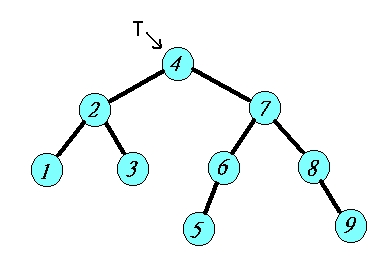Range Searching (Multi-key searching)Find Record = (X1,...,XN), s.t. , X1　 belong to [a1,b1] ↑ X2　 belong to [a2,b2] attribute • • • XN　 belong to [aN,bN]

 N = 1a1 = b1 : internal searchingPreprocessing
Range-SearchMethod 1

 Preprocessing : sorting N logN Range-Search : binary search R + logN
 Keys : 1 , 2 , 3 , 4 , 5 , 6 , 7 , 8 , 9 Q = [2.5,7] ↑ ↑Method 2
 Preprocessing : binary search tree N logN Range-Search : Search(l,[a,b]) R + logN

 if a <= l.key then search (l.left , [a,b]); if l.key <= b then search (l.right , [a,b]); if a <= l.key <= b then print (l.key);Q = [2.5 , 7]